# Trigonometry : Pythagorean Identities

## Example Questions

2 Next →

### Example Question #11 : Trigonometry

Reduce the following expression.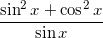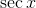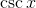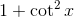Explanation:

Because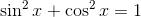therefore: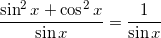By definition of cosecant,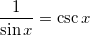### Example Question #21 : Trigonometric Identities

Reduce the following expression.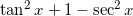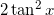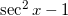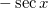Explanation:

There are several ways to work this problem, but all of them use the second Pythagorean trig identity,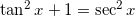.

You can use this identity to substitute for parts of the expression. Here are two examples.

Method 1:

Substituting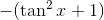for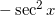, we get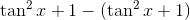, which equals zero.

Method 2:

Substituting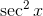for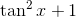, we get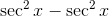, which equals zero.

Regardless of which substitution you choose, the answer is the same.

2 Next →

### All Trigonometry Resources